## Basic Low Voltage Regulator using IC 723:

Fig. 2.112 shows the Basic Low Voltage Regulator using IC 723.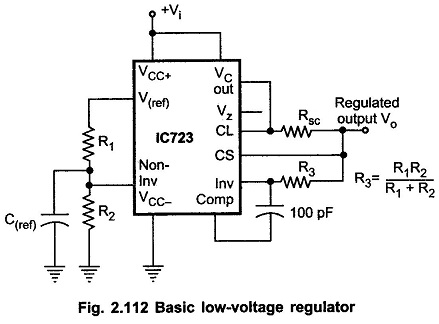The resistor, Rsc is connected between CL and CS pins. The current limit transistor remains non-conductive unless drops across Rsc is 0.6 V (equal to VBE drop). The value of Rsc can be found out by following equation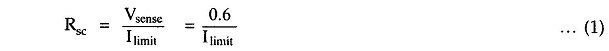Ilimit can be selected as 1.2 to 1.5 times the maximum load circuit. Potential divider made up of R1 and R2 is connected between Vref and non-inverting terminals.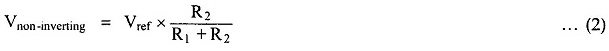As the series pass transistor is working as emitter follower.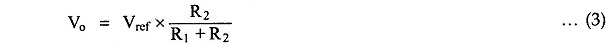R1 and R2 can be between 1 kΩ to 10 kΩ.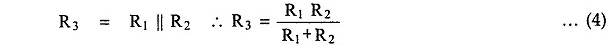Maximum load current can be 150 mA.

Scroll to Top